# Raspberry Pi Embedded Cap With GPS & 10DOF

In this post we will show you how to geotag and capture the "attitude"  of photos taken with the Raspberry Pi camera and record these values within the photo itself using EXIF metadata

We used a modified (hacked?) cap to take the images in this post. The cap took photos, geo-tagged and recorded attitude as we walked around Sydney Harbour.

Components used were;

• Raspberry Pi Zero W
• BerryGPS-IMU
• Raspberry Camera V2
• A cap

The BerryGPS-IMU was used to capture the GPS coordinates as well as "attitude".   No external antenna was needed as the BerryGPS-IMU includes an internal antenna.

The "attitude" would include values such as pitch, roll, direction. Some of this data you can see annotate in the image below.Other programs can use some of this data to plot the image on a map and even show the direction of the camera at the time the image was taken.  A good example of this is seen in  GeoSetter# The Cap

The cap has the BerryGPS-IMU sitting on top of the visor, with the Raspberry Pi sitting under the viso.  Some holes where made in the visor to allow connectivity between the BerryGPS-IMU and Raspberry Pi.
We also created a basic camera mount out of 3mm laser cut acrylic. M2.5 Nylon screws were used to hold everything in place.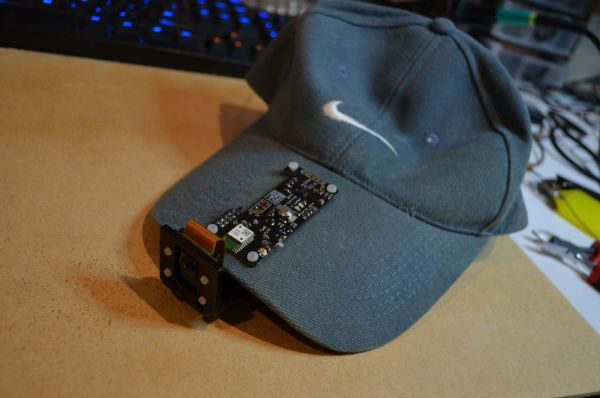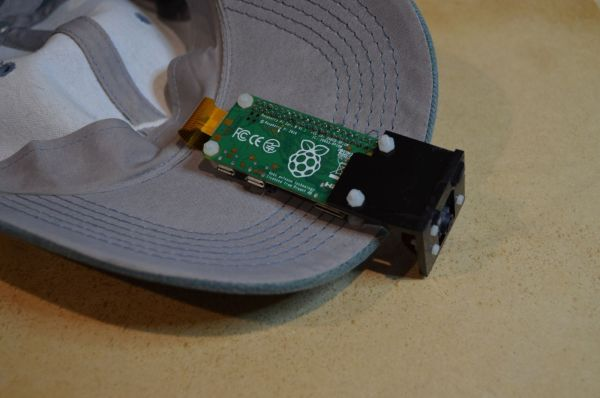# BerryIMU running on Raspberry Pi running Windows IoT

BerryIMU also works great with Windows IoT Core on the Raspberry Pi.

Our Git repository contains the source files needed to get the BerryIMU up and running on Windows IoT.

The code will print out the following values to the screen;

• Raw values from the gyroscope, accelerometer and magnetometer.
• Accelerometer calculated angles.
• Gyro tracked angles.
• Fused X and Y angles.

## Connecting BerryIMU to a Raspberry Pi

BrryIMU can connect via the jumper cables to the Raspberry Pi as shown below;Or BerryIMU can sit right on top of the GPIO pins on a Raspberry Pi. The first 6 GPIOs are used as shown below.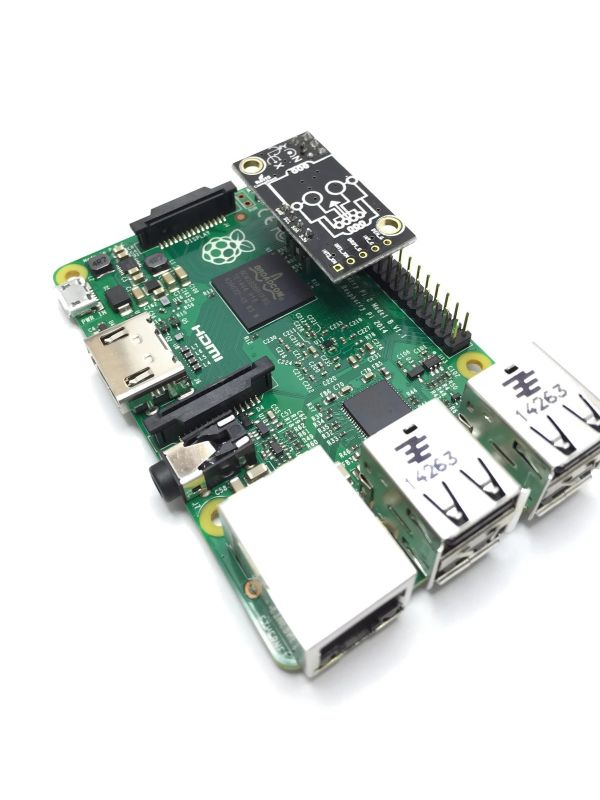# Get the Code

Download the BerryIMU code for Windows IoT from our GIT repository. The files you need are under the WindowsIoT-BerryIMU folder.

Once downloaded, double-click  the file WindowsIoT-BerryIMU.sln to open up the project in Visual Studio.

The project code outputs all of the needed values to the screen and a complementary filter is used to fuse the accelerometer and gyroscope angles.

We have a number of guides already documented on how to get the BerryIMU working with the Raspberry Pi.
https://ozzmaker.com/berryimu/
These are based on Raspbian, however the principals and math are the same for Windows Iot.

The final values which should be used are the fused X &Y angles and the tilt compensated heading.

The sensor on the BerryIMU is the LSM9DS0 and all the I2C registers for this sensor can be found in LSM9DS0.cs

The main code can be found in MainPage.xaml.cs#### Complementary Filter

A complementary filter is used to fuse the angles. Is summary, the complementary filter trusts the gyroscope for short periods and trusts the accelerometer for longer periods;

```CFangleX = AA * (CFangleX + (rate_gyr_x * DT / 1000)) + (1.0f - AA) * AccXangle;
CFangleY = AA * (CFangleY + (rate_gyr_y * DT / 1000)) + (1.0f - AA) * AccYangle;
```

Changing how much trust is given for each of the sensors can be changed by modify the complementary filter constant at the start of the code.

```const float AA = 0.03f;     // Complementary filter constant
```

#### Loop Speed

The loop speed is important as we need to know how much time has past to calculate the rotational degrees per second on the gyroscope.
A time delta is set at the start of the code.

```const int DT = 100;         //DT is the loop delta in milliseconds.
```

This is then used to specify a new timer method.

```periodicTimer = new Timer(this.TimerCallback, null, 0,DT);
```

Here you can see where DT is used to keep track of the gyroscope angle. You can also see it in the above calculation for the complementary filter.

```//Calculate the angles from the gyro
gyroXangle += rate_gyr_x * DT / 1000;
gyroYangle += rate_gyr_y * DT / 1000;
gyroZangle += rate_gyr_z * DT / 1000;
```

#### BerryIMU orientation

The calculations in the code are based on how the BerryIMU is orientated. If BerryIMU is upside down, then some of the angles need to be reversed. It is upside down when the skull logo is facing up(or to the sky).
If it is upside down, set the below value to true. Otherwise, set it to false.

```bool IMU_upside_down = true;
```

.

# BerryIMU Code for Teensy

Our BerryIMU GIT repository has been updated with code for the Teensy, specifically Teensy 3.6.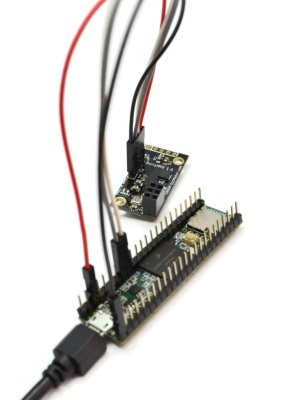Now you can have access to an accelerometer, gyroscope, compass, temperature and pressure sensor on your Teensy.

Here you can see the angles displayed using the Serial Plotter in the Arduino IDE which is connected to a Teensy 3.6.

# Teensy 3.6  HookupConnect SCL on the BerryIMU to PIN 19
Connect SDA on the BerryIMU to PIN 18
Use 3.3v pin on the Teensy to power the BerryIMU.

# Code

Download the BerryIMU code from our GIT repository. The files you need are under the Teensy-BerryIMU folder.

TeenysDuino
We use the Arduino IDE to program the Teensy.  You will need to install Teensduino so that your Arduino IDE supports Teensy.# Arduino Serial plotter

The latest version of Arduino IDE includes a Serial Plotter. This is great to show angles in a sliding graph.

The below image shows how to access the Serial Plotter.The Serial Monitor will have to be closed as both cannot be opened at the same time.

The Serial Plotter expects the values to be separated with a space. To show the X and Y angles on the plotter, comment out the print statements in the existing code and insert the code below.

```  Serial.print(AccXangle);
Serial.print("\t");
Serial.print(AccYangle);
```

# Record Temperature and Pressure with ESP8266 and BerryIMU

In this guide, we will be using the ESP8266 and BerryIMU to record temperature and pressure and display the output onto a web page.

Google charts is used to display the data in an easy to read format.

Components used are;

# Summary

• NTP is used to get the correct time of day.
• The free available RAM is calculated to work out how much data we can store,
• Google charts is used to display chart data.
• 4 web pages are created:
"/chart.html" - Is used to display chart.
"/table.html" - Is used to display the data in a table.
"/data.json"- Is used by the home page to update the the gauge values.

# Hook Up

The below diagram shows how to connect a BerryIMU to an ESP8266 microcontroller, in this case it is the  Adafruit Feather Huzzah .

# Prepare Arduino IDE

The Arduino IDE needs to be updated with the board packages for the ESP8266.  This is very easy to do and both Sparkfun & Adafruit have detailed guides on how to do this;
Sparkfun Arduino IDE and ESP8266

# ESP8266 and a BerryIMU - simple web server

The ESP8266 is another good microcontroller which can be used with the BerryIMU.

The ESP8266 is small and includes Wifi.

In this guide we will setup of the ESP8266 to provide a web page which we can then use to read the  accelerometer, gyroscope and compass values from the BerryIMU. We will also force this webpage to refresh every 1 seconds.

The ESP8266 Arduino core to program our ESP8266. This allows you to use the Arduino IDE to program and upload to the ESP8266.

We have used the Adafruit Feather Huzzah and the Sparkfun Thing Dev board in this guide. Both are excellent boards with included USB to serial converters. Just plug in and upload. This guide can also be used with other ESP8266 boards, just take note of the pins used.

# Hook Up

The below diagrams show how to connect a BerryIMU to an ESP8266 microcontroller, in this case the  Adafruit Feather Huzzah and the Sparkfun Thing Dev board.

# Prepare Arduino IDE

The Arduino IDE needs to be updated with the board packages for the ESP8266.  This is very easy to do and both Sparkfun & Adafruit have detailed guides on how to do this;
Sparkfun Arduino IDE and ESP8266

# The Code

The code can be found here . Download the entire Git repo. The code for this guide can be found under the directory
ESP8266-BerryIMU/BerryIMU_ESP8266_simple_web/
The file you load into the Arduino IDE is BerryIMU_ESP8266_simple_web.ino.

This guide will only cover the specific to the ESP8266. There is another guide here https://ozzmaker.com/berryimu/ which covers the code used to calculate the angles and heading from the BerryIMU.

The first thing to do is update the code with your wireless network settings.

```const char* ssid = "******";
```

Further down you can see where we define the web server and what port to listen on

```ESP8266WebServer server(80);
```

There is then a function called handleroot(). This is what builds the web page and sends it to the client when the client requests it E.g. When a web browser requests for a page.
Looking at the line which contains the meta tag, you can see where the refresh timer is set to 1 seconds.

I have also hilighted the variables which store the angles and heading from the BerryIMU.

```void handleroot()
{
//Create webpage with BerryIMU data which is updated every 1 seconds
server.sendContent("HTTP/1.1 200 OK\r\n"); //send new p\r\nage
server.sendContent("Content-Type: text/html\r\n");
server.sendContent("\r\n");
server.sendContent
"<h3 style=text-align:center;font-size:200%;color:RED;>BerryIMU and ESP8266</h3>"
"<h3 style=text-align:center;font-size:100%;>accelerometer, gyroscope, magnetometer</h3>"
"<h3 style=text-align:center;font-family:courier new;><a href=https://ozzmaker.com/ target=_blank>https://ozzmaker.com</a></h3><hr>");
server.sendContent
("<h2 style=text-align:center;> Filtered X angle= " + String(<strong><span style="color: #ff0000;">CFangleX</span></strong>));
server.sendContent
("<h2 style=text-align:center;> Filtered Y angle= " + String(<span style="color: #ff0000;"><strong>CFangleY</strong></span>));
server.sendContent
server.sendContent
}
```

Within setup(), we define what pins are used for I2C to communicated with the BerryIMU.

```Wire.begin(4,5);
```

The first value is the SDA pin and the second specifies the SCL pin. Any pin on the ESP8266 can be used for I2C.

Wireless is then enabled  and then we try and connect to the wireless network. The IP address is then printed to the serial console.

```  WiFi.begin(ssid, password);

// Wait for connection
while (WiFi.status() != WL_CONNECTED)
{
delay(500);
Serial.print(".");
}
Serial.println("");
Serial.print("Connected to ");
Serial.println(ssid);

//Print IP to console
Serial.println(WiFi.localIP());
delay(3);
```

And finally, the web server is started and match on root of the web server and then run handleroot().

```server.on("/", handleroot);
```

You need to add the below line in the main loop to handle web requests.

```server.handleClient(); //Handler for client connections
```

# Converting values from an Accelerometer to G

In this post I will show how to convert the raw values read from an accelerometer to 'Gs'.

Git repository here
The code can be pulled down to your Raspberry Pi with;

pi@raspberrypi ~ \$ git clone https://github.com/ozzmaker/BerryIMU.git

The code for this guide can be found under the python-BerryIMU-measure-G directory.

An accelerometer measures proper acceleration, which is the acceleration it experiences relative to freefall. This is most commonly called "G-Force" (G)

For example, an accelerometer at resting on a table would measure 1G ( 9.81 m/s2) straight upwards. By contrast, accelerometers in free fall and accelerating due to the gravity of Earth will measure zero.

The accelerometer used by the BerryIMU is a MEMS sensors(LSM9DS0), which outputs the raw readings as mg/LSB.
Most MEMS accelerometers use this output format.

mg = milli-G's (just like milliliters)
1mG = 0.001 G's of acceleration, so 1000mG = 1G.
LSB = Least Significant bit, which is the last bit on the right.The LSM9DS0 outputs a 16 bit value for the accelerometer readings.

If you look at the characteristics of the LSM9DS0 in the datasheet, you can see the sensitivity levels for the accelerometer highlighted in red below and the corresponding values for the LSB, which are highlighted in blue. You can download the datasheet here;

The raw values from the accelerometer are  multiplied by the sensitive level to get the value in G.Let's use FS ±2 g as an example sensitivity level.  As the range is -2 to +2, this would be a total of 4g.  Or 4,000 Milli-Gs.
The output is 16 bits. 16 bits equals 65,535.   This means we can get 65,535 different readings for the range  between -2 and +2. (or -2,000 MilliGs and +2,000 MilliGs)

4,000 MilliGs / 65,535 = 0.061

Each time the LSB changes by one, the value changes by 0.061, which is the value highlighted in blue in the table above.

For FS ±8 g, the range would be -8 to +8, which is a total of 16,000 MilliGs.
16,000 MilliGs / 65,535 = 0.244

Example when using ±2g sensitivity
In the table below, every time the raw values increments by one, the final calculated value(which is MilliG) increments by 0.061

```RAW  	BINARY	LSB value for +/-2G	Calc MilliG
16	10000		0.061		0.976
17	10001		0.061		1.037
18	10010		0.061		1.098```

The above values of 16,17 and 18 above a very low and only used for illustration.
If your accelerometer is horizontal and resting and at rest when using a sensitive level of ±2g, the raw value for Z should hover  around 16,500.
16,500 X 0.061 = 1006 MilliGs or 1G

Example when using ±8g sensitivity
In the table below, every time the raw values increments by one, the final calculated value(which is MilliG) increments by 0.244

```RAW  	BINARY	LSB value for +/-2G	Calc MilliG
16	10000		0.244		3.904
17	10001		0.244		4.148
18	10010		0.244		4.392```

If you accelerometer is horizontal and at rest, when using a sensitive level of ±8g, the raw value for Z should hover  around 4,475.

4,175
X 0.244 = 1018.7 MilliGs or 1G

# The Code

The two above examples are easy to implement in python;
±8g Sensitivity

```writeACC(CTRL_REG2_XM, 0b00010000) #+/- 8G full scale
print("G Value for Z axis %f G" % ((ACCz * 0.244)/1000))
```

The first line above is used to initialise the accelerometer with a sensitivity level of ±2g.
The second line prints the calculated value as G using the raw values from the accelerometer.

±2g Sensitivity

```writeACC(CTRL_REG2_XM, 0b00000000) #+/- 2G full scale
print("G Value for Z axis %f G" % ((ACCz * 0.061)/1000))
```

The first line above is used to initialise the accelerometer with a sensitivity level of ±2g.
The second line prints the calculated value as G uses using raw values from the accelerometer.
Below is a snippet from the main program;

```import smbus
import time
import math
from LSM9DS0 import *
import datetime
bus = smbus.SMBus(1)

def writeACC(register,value):
return -1

acc_combined = (acc_l | acc_h <<8)

return acc_combined  if acc_combined < 32768 else acc_combined - 65536

acc_combined = (acc_l | acc_h <<8)

return acc_combined  if acc_combined < 32768 else acc_combined - 65536

acc_combined = (acc_l | acc_h <<8)

return acc_combined  if acc_combined < 32768 else acc_combined - 65536

#initialise the accelerometer
writeACC(CTRL_REG1_XM, 0b01100111) #z,y,x axis enabled, continuos update,  100Hz data rate
writeACC(CTRL_REG2_XM, 0b00011000) #+/- 8G full scale

while True:

#Read the accelerometer,gyroscope and magnetometer values

print("##### X = %f G  #####" % ((ACCx * 0.244)/1000)),
print(" Y =   %fG  #####" % ((ACCy * 0.244)/1000)),
print(" Z =  %fG  #####" % ((ACCz * 0.244)/1000))

#slow program down a bit, makes the output more readable
time.sleep(0.03)
```

# Raspberry Pi Digital Spirit Level

In this post we show how to create a Digital Spirit Level using a Raspberry Pi and python.

The code moves that bubbles on the display in relation to the angle read from the IMU.
Parts used in this project;

Any IMU or TFT can be used, however the code would need to be updated to accommodate the different devices. It is best to use a 480x320 TFT as the images are scaled to fit this resolution.

This guide assumes that some basic understanding of an IMU(Accelerometer and Gyroscope)  is already known. And you have one already working with your Raspberry Pi.

If you don't, we do have some guides which covers this.We have used our existing python code to read the values from the IMU, however we have removed the code related to the magnetometer as it isn't needed for this project.

Git repository here
The code can be pulled down to your Raspberry Pi with;

pi@raspberrypi ~ \$ git clone http://github.com/ozzmaker/BerryIMU.git

## Placement of IMU

The IMU can be attached anywhere, however it is best to place it in the same orientation as shown below. If you do change the orientation, you will need to update the code accordingly.

# BerryIMU Python Code Update - Kalman Filter and More

We have updated to the python code in our git repo.

It now includes;

• The elusive Kalman filter.
• Math needed when the IMU is upside down
• Automatically calculate loop period.

What is a Kalman filter?  In a nutshell;
A Kalman filter is, it is an algorithm which uses a series of measurements observed over time, in this context an accelerometer and a gyroscope. These measurements will contain noise that will contribute to the error of the measurement. The Kalman filter will then try to estimate the state of the system, based on the current and previous states, that tend to be more precise that than the measurements alone.

A Kalman filter is more precise than a Complementary filter. This can be seen in the image below, which is the output of a complementary filter (CFangleX) and a Kalman filter (kalmanX) from the X axis plotted in a graph.

The red line (KalmanX) is better at filtering out noisep;The code can be found here in our Git repository here
And  can be pulled down to your Raspberry Pi with;

pi@raspberrypi ~ \$ git clone http://github.com/ozzmaker/BerryIMU.gitA summary of the code;

```
def kalmanFilterY ( accAngle, gyroRate, DT):
y=0.0
S=0.0

global KFangleY
global Q_angle
global Q_gyro
global y_bias
global XP_00
global XP_01
global XP_10
global XP_11
global YP_00
global YP_01
global YP_10
global YP_11

KFangleY = KFangleY + DT * (gyroRate - y_bias)

YP_00 = YP_00 + ( - DT * (YP_10 + YP_01) + Q_angle * DT )
YP_01 = YP_01 + ( - DT * YP_11 )
YP_10 = YP_10 + ( - DT * YP_11 )
YP_11 = YP_11 + ( + Q_gyro * DT )

y = accAngle - KFangleY
S = YP_00 + R_angle
K_0 = YP_00 / S
K_1 = YP_10 / S

KFangleY = KFangleY + ( K_0 * y )
y_bias = y_bias + ( K_1 * y )

YP_00 = YP_00 - ( K_0 * YP_00 )
YP_01 = YP_01 - ( K_0 * YP_01 )
YP_10 = YP_10 - ( K_1 * YP_00 )
YP_11 = YP_11 - ( K_1 * YP_01 )

return KFangleY

```

# BerryIMU and Pi Zero

BerryIMU is a perfect companion for Pi Zero.

We have confirmed that BerryIMU is 100% compatible with the Pi Zero. BerryIMU can sit right on top of Pi Zero header pins while still maintaining a very low profile.# BerryIMU code for Arduino - Accelerometer, Gyroscope and Magnetometer

Our GIT repository has been updated with an Arduino sketch which calculates angles using a complementary filter.  The heading is also calculated using the magnetometer.

#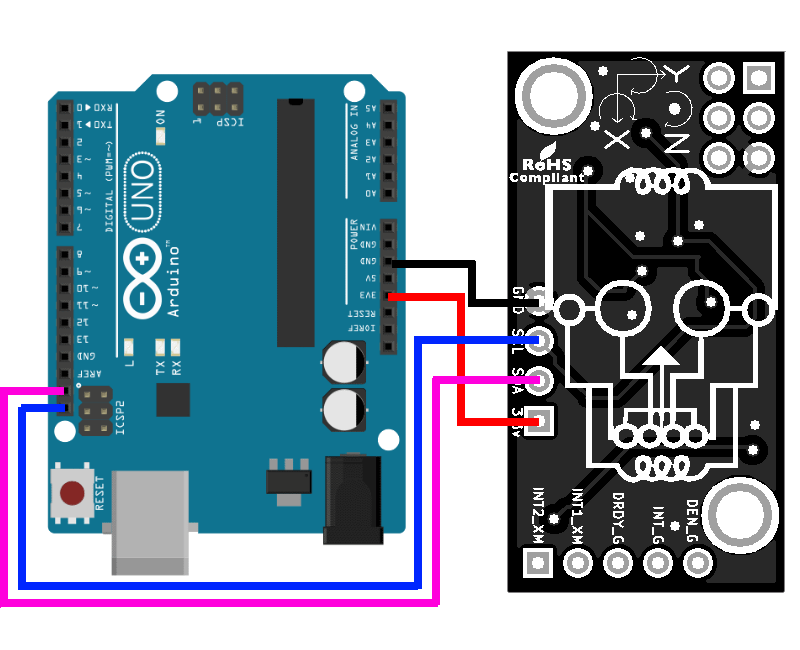## Detailed Guides and Tutorials

These cover both BerryIMU and displaying graphics with SDL on a Raspberry Pi;
How to Create an Inclinometer using a Raspberry Pi and an IMU